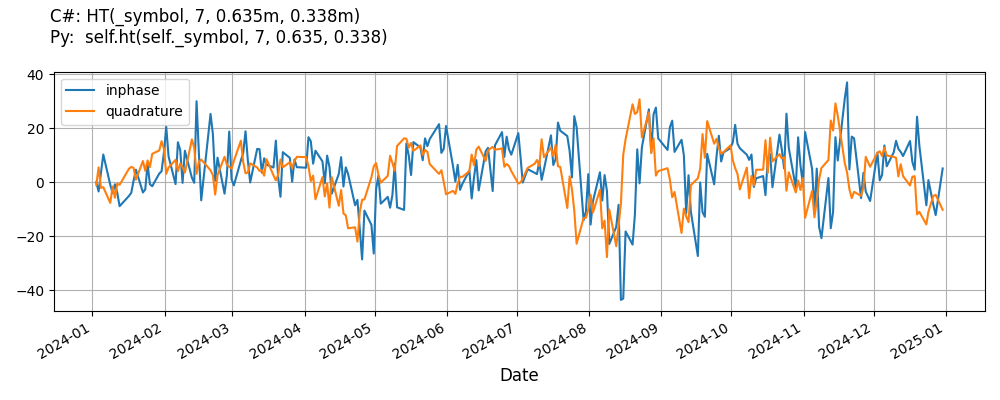# Supported Indicators

## Hilbert Transform

### Introduction

This indicator computes the Hilbert Transform Indicator by John Ehlers. By using present and prior price differences, and some feedback, price values are split into their complex number components of real (inPhase) and imaginary (quadrature) parts. source

To view the implementation of this indicator, see the LEAN GitHub repository.

### Using HT Indicator

To create an automatic indicators for HilbertTransform, call the HT helper method from the QCAlgorithm class. The HT method creates a HilbertTransform object, hooks it up for automatic updates, and returns it so you can used it in your algorithm. In most cases, you should call the helper method in the Initialize method.

public class HilbertTransformAlgorithm : QCAlgorithm
{
private Symbol _symbol;
private HilbertTransform _ht;

public override void Initialize()
{
_ht = HT(_symbol, 7, 0.635, 0.338);
}

public override void OnData(Slice data)
{
{
// The current value of _ht is represented by itself (_ht)
// or _ht.Current.Value
Plot("HilbertTransform", "ht", _ht);
// Plot all properties of ht
Plot("HilbertTransform", "inphase", _ht.InPhase);
}
}
}
class HilbertTransformAlgorithm(QCAlgorithm):
def Initialize(self) -> None:
self.ht = self.HT(self.symbol, 7, 0.635, 0.338)

def OnData(self, slice: Slice) -> None:
# The current value of self.ht is represented by self.ht.Current.Value
self.Plot("HilbertTransform", "ht", self.ht.Current.Value)
# Plot all attributes of self.ht
self.Plot("HilbertTransform", "inphase", self.ht.InPhase.Current.Value)


The following reference table describes the HT method:

### HT()1/1

            HilbertTransform QuantConnect.Algorithm.QCAlgorithm.HT (
Symbol                           symbol,
Int32                            length,
Decimal                          inPhaseMultiplicationFactor,
Decimal                          quadratureMultiplicationFactor,
*Nullable<Resolution>      resolution,
*Func<IBaseData, Decimal>  selector
)


Creates a new Hilbert Transform indicator.

If you don't provide a resolution, it defaults to the security resolution. If you provide a resolution, it must be greater than or equal to the resolution of the security. For instance, if you subscribe to hourly data for a security, you should update its indicator with data that spans 1 hour or longer.

You can manually create a HilbertTransform indicator, so it doesn't automatically update. Manual indicators let you update their values with any data you choose.

Updating your indicator manually enables you to control when the indicator is updated and what data you use to update it. To manually update the indicator, call the Update method with time/number pair, or an IndicatorDataPoint. The indicator will only be ready after you prime it with enough data.

public class HilbertTransformAlgorithm : QCAlgorithm
{
private Symbol _symbol;
private HilbertTransform _ht;

public override void Initialize()
{
_ht = new HilbertTransform(7, 0.635, 0.338);
}

public override void OnData(Slice data)
{
if (data.Bars.TryGeValue(_symbol, out var bar))
{
_ht.Update(bar.EndTime, bar.Close);
}

{
// The current value of _ht is represented by itself (_ht)
// or _ht.Current.Value
Plot("HilbertTransform", "ht", _ht);
// Plot all properties of ht
Plot("HilbertTransform", "inphase", _ht.InPhase);
}
}
}
class HilbertTransformAlgorithm(QCAlgorithm):
def Initialize(self) -> None:
self.ht = HilbertTransform(7, 0.635, 0.338)

def OnData(self, slice: Slice) -> None:
bar = slice.Bars.get(self.symbol)
if bar:
self.ht.Update(bar.EndTime, bar.Close)

# The current value of self.ht is represented by self.ht.Current.Value
self.Plot("HilbertTransform", "ht", self.ht.Current.Value)
# Plot all attributes of self.ht
self.Plot("HilbertTransform", "inphase", self.ht.InPhase.Current.Value)


To register a manual indicator for automatic updates with the security data, call the RegisterIndicator method.

public class HilbertTransformAlgorithm : QCAlgorithm
{
private Symbol _symbol;
private HilbertTransform _ht;

public override void Initialize()
{
_ht = new HilbertTransform(7, 0.635, 0.338);
RegisterIndicator(_symbol, _ht, Resolution.Daily);
}

public override void OnData(Slice data)
{
{
// The current value of _ht is represented by itself (_ht)
// or _ht.Current.Value
Plot("HilbertTransform", "ht", _ht);
// Plot all properties of ht
Plot("HilbertTransform", "inphase", _ht.InPhase);
}
}
}
class HilbertTransformAlgorithm(QCAlgorithm):
def Initialize(self) -> None:
self.ht = HilbertTransform(7, 0.635, 0.338)
self.RegisterIndicator(self.symbol, self.ht, Resolution.Daily)

def OnData(self, slice: Slice) -> None:
# The current value of self.ht is represented by self.ht.Current.Value
self.Plot("HilbertTransform", "ht", self.ht.Current.Value)
# Plot all attributes of self.ht
self.Plot("HilbertTransform", "inphase", self.ht.InPhase.Current.Value)


The following reference table describes the HilbertTransform constructor:

### HilbertTransform()1/2

            HilbertTransform QuantConnect.Indicators.HilbertTransform (
string  name
)


Creates a new Hilbert Transform indicator.

### HilbertTransform()2/2

            HilbertTransform QuantConnect.Indicators.HilbertTransform (

)


Creates a new Hilbert Transform indicator with default name and default params.

### Visualization

The following image shows plot values of selected properties of HilbertTransform using the plotly library.You can also see our Videos. You can also get in touch with us via Discord.